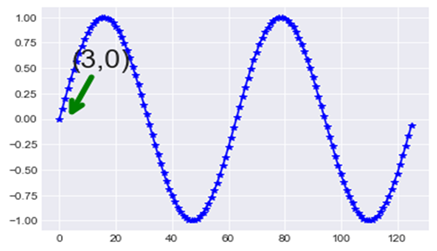# How to add annotations in Matplotlib Plots?

To specify the details of a plot, we use annotations. To create annotations in Matplotlib Plots, we can use the ‘annotate’ method.

## Example

import matplotlib.pyplot as plt
import numpy as np
#Let us create a plot and use annotation at the point (5,3),
x = np.arange(0,4*np.pi,0.1)
plt.plot(np.sin(x), 'b-*')
a = plt.annotate("(3,0)", xy=(3, 0), xycoords='data',
xytext=(4.0,0.5), textcoords='data',
arrowprops=dict(arrowstyle="->", color="green", lw=5,
plt.show()